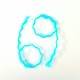69 Meme Internet Sensation

69 meme became an internet sensation because WWW (World Wide Web) is itself a meme. 69 and meme are essentially tao symbol of the Internet. Browsing the Internet is 69ing.

In 69 (Tao), 6 and 9 are yin and yang.
6 = Yin
9 = Yang

6 is the number of square faces in a Rubik’s Cube and 9 is the number of sub-squares per square face. There are 54 sub-squares in a Rubik’s Cube.

69 (Tao) = Yin and Yang
= 6 and 9
= 6 × 9
= 54

54 is Love, which means that the World Wide Web is Love.

Love = L + O + V + E
= 12 + 15 + 22 + 5
= 54

69 meme is 69 tao, because tao and meme are numerically the same thing.

Tao = T + O + A
= 20 + 15 + 1
= 36

Meme = M + E + M + E
= 13 + 5 + 13 + 5
= 18 + 18
= 36 (Tao)

69 is a lucky number because good luck is a life force called chi or qi.

The lucky number is the winning number.

Win = W

W is 23, the 23rd letter of the English alphabet.

WWW (World Wide Web) is 69.

WWW = 3W
= 3 × W
= 3 × 23
= 69 (Tao)

Without “nice” the 69 meme is incomplete. That is why people usually reply “nice” to posts containing 69.

Nice = N + I  + C + E
= 14 + 9 + 3 + 5
= 31

69 + Nice = 69 + 31
= 100%

Note that the letters of the English alphabet are numbered 1 to 26. Examples: A = 1, B = 2, O = 15, T = 20, and Z = 26.

1.Gottfried says:

Liked by 1 person

•69math says:

Alright

Liked by 1 person

•Gottfried says:

You have a few more to go 😊

Liked by 1 person

•69math says:

Nice

Liked by 1 person

•Gottfried says:

You done now?

Liked by 1 person

•69math says:

Yeah, am done for the day.

Liked by 1 person

•Gottfried says:

C’mon, just a few hundred to go 😅

Liked by 1 person

•69math says:

Lol

Liked by 1 person

•Gottfried says:

Liked by 1 person

•69math says:

Those hundreds should know that I will certainly pay a visit.

Liked by 1 person

•Gottfried says:

haha, you’re getting close

Liked by 1 person

•69math says:

I have exceeded 100

Liked by 1 person

•Gottfried says:

haha, a little way off the finish line now

Liked by 1 person

•69math says:

•Gottfried says: